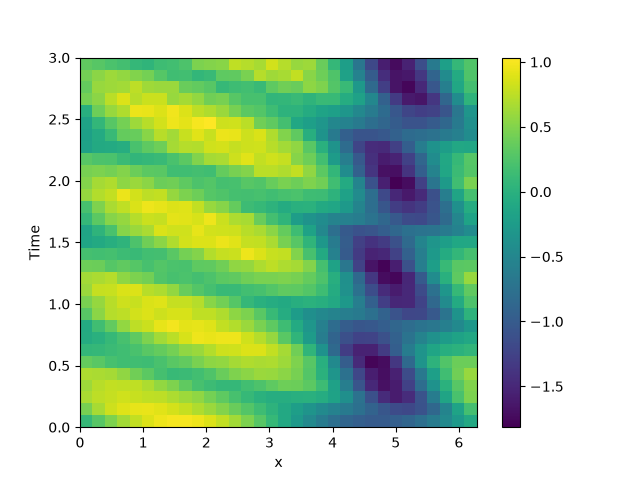# 2.12. 1D problem - Using PDE class¶

This example implements a PDE that is only defined in one dimension. Here, we chose the Korteweg-de Vries equation, given by

$\partial_t \phi = 6 \phi \partial_x \phi - \partial_x^3 \phi$

which we implement using the PDE.from math import pi

from pde import PDE, CartesianGrid, MemoryStorage, ScalarField, plot_kymograph

# initialize the equation and the space
eq = PDE({"φ": "6 * φ * d_dx(φ) - laplace(d_dx(φ))"})
grid = CartesianGrid([[0, 2 * pi]], , periodic=True)
state = ScalarField.from_expression(grid, "sin(x)")

# solve the equation and store the trajectory
storage = MemoryStorage()
eq.solve(state, t_range=3, tracker=storage.tracker(0.1))

# plot the trajectory as a space-time plot
plot_kymograph(storage)


Total running time of the script: ( 0 minutes 6.897 seconds)

Gallery generated by Sphinx-Gallery